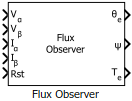# Flux Observer

Compute electrical position, magnetic flux, and electrical torque of rotor

• Library:
• Motor Control Blockset / Sensorless Estimators

•## Description

The Flux Observer block computes the electrical position, magnetic flux, and electrical torque of a PMSM by using the per unit voltage and current values along the α- and β-axes in the stationary αβ reference frame.

### Equations

These equations describe the computation of the electrical position, magnetic flux, and electrical torque by the block.

`${T}_{\text{e}}=\frac{3}{2}P\left({\psi }_{1\alpha }{I}_{\beta }-{\psi }_{1\beta }{I}_{\alpha }\right)$`

where:

• ${V}_{\alpha }$ and ${V}_{\beta }$ are the α-axis and β-axis voltages.

• ${I}_{\alpha }$ and ${I}_{\beta }$ are the α-axis and β-axis current.

• $R$ is the stator resistance of the motor.

• $P$ is the number of motor pole pairs.

• $\psi$ is the magnetic stator flux.

• ${T}_{e}$ is the electrical torque of the rotor.

• ${\theta }_{e}$ is the electrical position of the rotor.

## Ports

### Input

expand all

Voltage component along the α-axis in the stationary αβ reference frame.

Data Types: `single` | `double` | `fixed point`

Voltage component along the β-axis in the stationary αβ reference frame.

Data Types: `single` | `double` | `fixed point`

Current along the α-axis in the stationary αβ reference frame.

Data Types: `single` | `double` | `fixed point`

Current along the β-axis in the stationary αβ reference frame.

Data Types: `single` | `double` | `fixed point`

The pulse (true value) that resets the block algorithm.

Data Types: `single` | `double` | `fixed point`

### Output

expand all

The electrical position of the rotor as estimated by the block.

#### Dependencies

To enable this port, set Block output to `Position`.

Data Types: `single` | `double` | `fixed point`

The magnetic flux of the rotor as estimated by the block.

#### Dependencies

To enable this port, set Block output to `Flux`.

Data Types: `single` | `double` | `fixed point`

The electrical torque of the rotor as estimated by the block.

#### Dependencies

To enable this port, set Block output to `Torque`.

Data Types: `single` | `double` | `fixed point`

## Parameters

expand all

### Motor parameters

Select the unit of the α and β-axes voltage and current input values.

Select one or more quantities that the block should compute and display in the block output.

### Note

You must select at least one value. The block displays an error message if you click or without selecting any value.

Number of pole pairs available in the motor.

#### Dependencies

To enable this parameter, set Block output to `Torque`.

Stator phase winding resistance of the motor in ohms.

The fixed time interval in seconds between two consecutive instances of block execution.

### Datatypes

Unit of the electrical position output.

#### Dependencies

To enable this parameter, set Block output to `Position`.

Data type of the electrical position output.

#### Dependencies

To enable this parameter, set Block output to `Position`.

Unit of the magnetic flux output.

#### Dependencies

To enable this parameter, set Block output to `Flux`.

Data type of the magnetic flux output.

#### Dependencies

To enable this parameter, set Block output to `Flux`.

Unit of the electrical torque output.

#### Dependencies

To enable this parameter, set Block output to `Torque`.

Data type of the electrical torque output.

#### Dependencies

To enable this parameter, set Block output to `Torque`.

 O. Sandre-Hernandez, J. J. Rangel-Magdaleno and R. Morales-Caporal, "Simulink-HDL cosimulation of direct torque control of a PM synchronous machine based FPGA," 2014 11th International Conference on Electrical Engineering, Computing Science and Automatic Control (CCE), Campeche, 2014, pp. 1-6. (doi: 10.1109/ICEEE.2014.6978298)

 Y. Inoue, S. Morimoto and M. Sanada, "Control method suitable for direct torque control based motor drive system satisfying voltage and current limitations," The 2010 International Power Electronics Conference - ECCE ASIA -, Sapporo, 2010, pp. 3000-3006. (doi: 10.1109/IPEC.2010.5543698)

##### SupportGet trial now